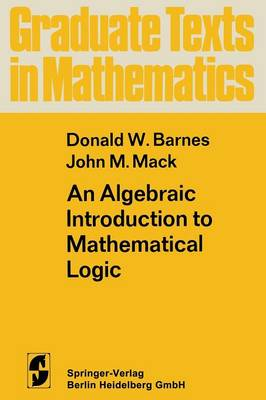•An Algebraic Introduction to Mathematical Logic - Graduate Texts in Mathematics 22 (Paperback)

(author), (author)
£59.99
Paperback 123 Pages / Published: 26/02/2013
• We can order this

Usually dispatched within 3 weeks

This book is intended for mathematicians. Its origins lie in a course of lectures given by an algebraist to a class which had just completed a sub-stantial course on abstract algebra. Consequently, our treatment of the sub-ject is algebraic. Although we assume a reasonable level of sophistication in algebra, the text requires little more than the basic notions of group, ring, module, etc. A more detailed knowledge of algebra is required for some of the exercises. We also assume a familiarity with the main ideas of set theory, including cardinal numbers and Zorn's Lemma. In this book, we carry out a mathematical study of the logic used in mathematics. We do this by constructing a mathematical model of logic and applying mathematics to analyse the properties of the model. We therefore regard all our existing knowledge of mathematics as being applicable to the analysis of the model, and in particular we accept set theory as part of the meta-Ianguage. We are not attempting to construct a foundation on which all mathematics is to be based--rather, any conclusions to be drawn about the foundations of mathematics come only by analogy with the model, and are to be regarded in much the same way as the conclusions drawn from any scientific theory.

Publisher: Springer-Verlag New York Inc.
ISBN: 9781475744910
Number of pages: 123
Weight: 219 g
Dimensions: 235 x 155 x 7 mm
Edition: Softcover reprint of the original 1st ed. 197